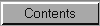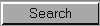Using Other Advanced Functions

To use the other advanced functions in the scientific calculator, click the following buttons or press the keyboard equivalent.
ButtonKeyFunction

cosoCalculates the cosine of the displayed number. Inv+cos calculates the arc cosine. Hyp+cos calculates the hyperbolic cosine. Inv+Hyp+cos calculates the arc hyperbolic cosine.
Deg
F2</fo